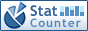### Vector-logic computing for faults-as-address deductive simulation

Wajeb Gharibi, Vladimir Hahanov, Svetlana Chumachenko, Eugenia Litvinova, Ivan Hahanov, Irina Hahanova

#### Abstract

The aim of the research is to create logic-free vector computing, leveraging read-write transactions in memory, to solve the problems of modeling and simulation stuck-at-fault combinations for complex logic elements and digital structures. At the same time, the problem of creating smart data structures based on logical vectors, truth tables, and deductive matrices is considered to simplify algorithms for parallel stuck-at-fault simulation. Vector computing is a computational process based on read-write transactions on bits of a binary vector of functionality, where the input data and faults are the addresses of the bits. A method for the synthesis of deductive vectors for propagating input fault lists is proposed, which has a quadratic computational complexity of read-write transactions. Deductive vectors, combined into a quadratic matrix, represent explicit data structures for parallel simulation of single and multiple stuck-at-faults. The initial information for constructing a deductive matrix is a logical vector and a bit-recoding matrix. Matrix is easily obtained using a recursive procedure based on the combinatorial properties of the truth table. Considering emerging trends, focused on in-memory computing, an algorithm for fault, as addresses, simulation is proposed, using logical and deductive vectors placed in memory. The simulation algorithm is proposed not to use commands of powerful processors.

#### Keywords

in-memory computing; logical vector; matrix of deductive vectors; read-write transaction; sequencer of vector deductive fault simulation; vector model of input faults;

#### Full Text:

PDF

DOI: http://doi.org/10.11591/ijra.v12i3.pp274-288

### Refbacks

• There are currently no refbacks.

IAES International Journal of Robotics and Automation (IJRA)
ISSN 2089-4856, e-ISSN 2722-2586
This journal is published by the Institute of Advanced Engineering and Science (IAES) in collaboration with Intelektual Pustaka Media Utama (IPMU).IJRA Visitor Statistics